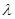Chapter 27, Problem 34CQ

Chapter
Section
Textbook Problem

When particles scattering light are smaller than its wavelength, the amount of scattering is proportional to 1 / λ 4 . Does this mean there is more scattering for small λ than largeHow does this relate to the fact that the sky is blue?

To determine

The reason for to pass some light through two perfect polarizing filter with perpendicular axes when a third polarizing filter is placed between them and the circumstances under which most of light pass.

Explanation

Introduction:

The Rayleigh scattering occurs when the particles causing the scattering are smaller in size than the wavelengths of radiation in contact with them.

Rayleigh scattering depends on the wavelength of light as,

Rayleighscattering1λ4

Thus, as the wavelength decreases, the amount of scattering increases

Still sussing out bartleby?

Check out a sample textbook solution.

See a sample solution

The Solution to Your Study Problems

Bartleby provides explanations to thousands of textbook problems written by our experts, many with advanced degrees!

Get Started